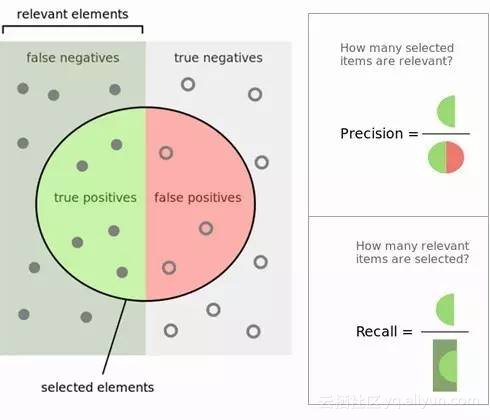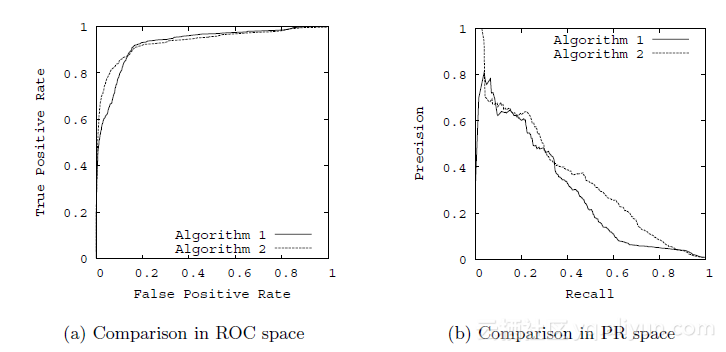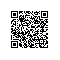# 精确率与召回率，RoC曲线与PR曲线

1. TP, FP, TN, FN

1. True Positives,TP：预测为正样本，实际也为正样本的特征数
2. False Positives,FP：预测为正样本，实际为负样本的特征数
3. True Negatives,TN：预测为负样本，实际也为负样本的特征数
4. False Negatives,FN：预测为负样本，实际为正样本的特征数2. 精确率(precision),召回率(Recall)与特异性(specificity)

$$P = \frac{TP}{TP + FP }$$

$$R = \frac{TP}{TP + FN }$$

$$S = \frac{TN}{FP + TN }$$

$$\frac{2}{F_1} = \frac{1}{P} + \frac{1}{R}$$

$$F_\beta = \frac{(1+\beta^2)*P*R}{\beta^2*P + R}$$

$$TPR = \frac{TP}{TP + FN }$$

$$FPR = \frac{FP}{FP + TN }$$

3. RoC曲线和PR曲线使用钉钉扫一扫加入圈子
+ 订阅